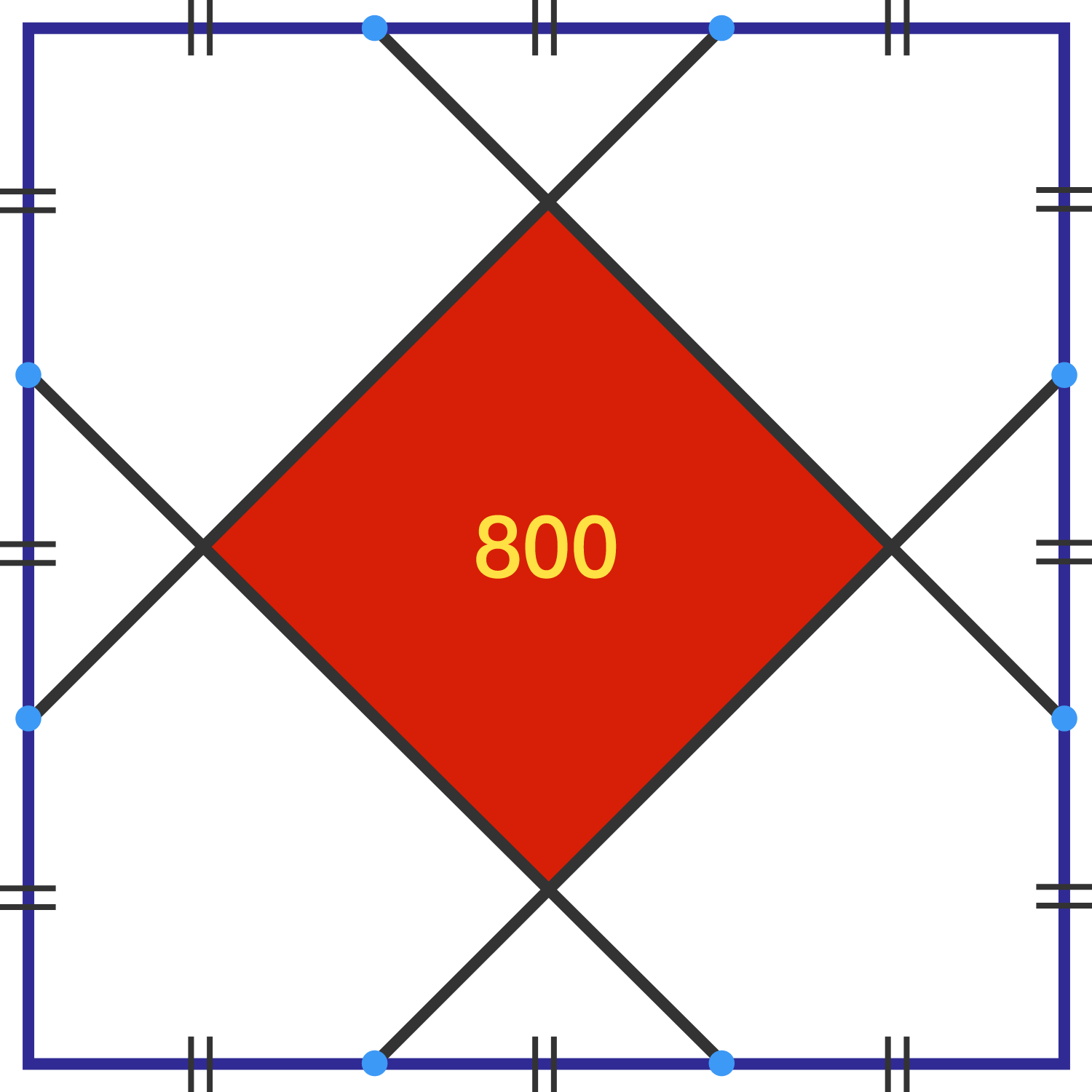# Tri

Geometry Level 1

Each side of a square is divided into three equal parts by two points on it. The points are then connected by line segments, as shown in the diagram below (that is not drawn to scale).

If the smaller, red square has an area of 800, what is the area of the large square?×